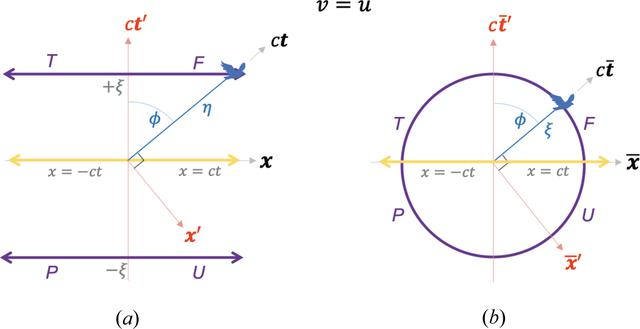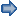disable zoom     view article Figure 9 A special case of the blended and the RBS coordinates, where v = u, and hence. (a) A plot of the blended coordinates given in equation (7a)with equation (8)substituted in. (b) RBS coordinates, given in equation (11b), transform (a) into a circle of radius, where the four hyperbola branches in (a) become four arc segments of the circle. Remarkably, all the arc segments now represent directions from the origin along which time-like events occur. Blended and RBS light lines are shown by the horizontal yellow line. See the Mathematica script in the Mathematica notebook in the supporting information to generate this plot.FOUNDATIONSADVANCES
ISSN: 2053-2733
Volume 77| Part 4| July 2021| Pages 242-256